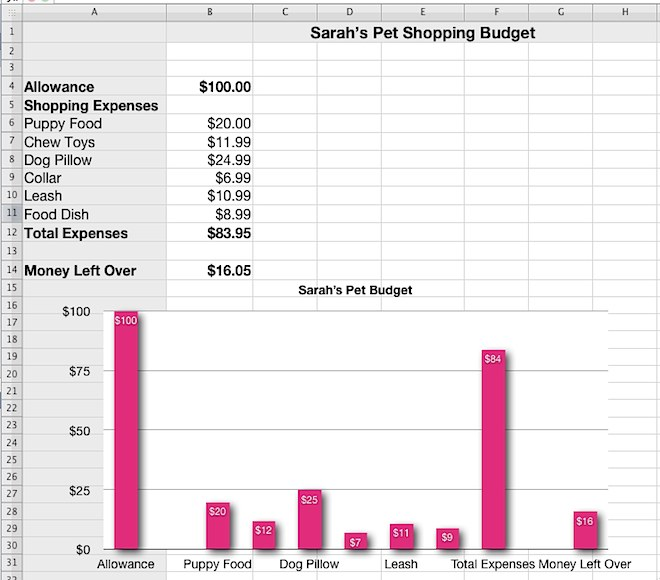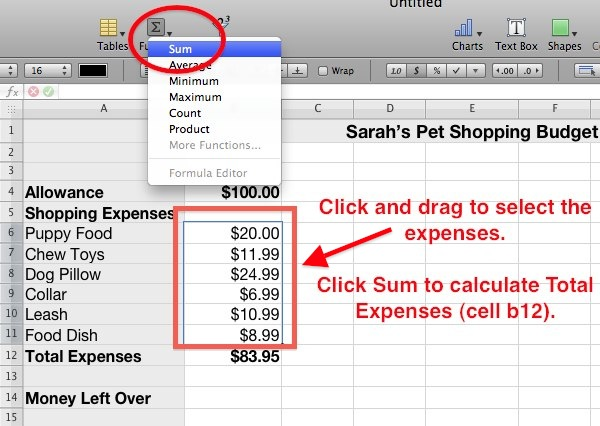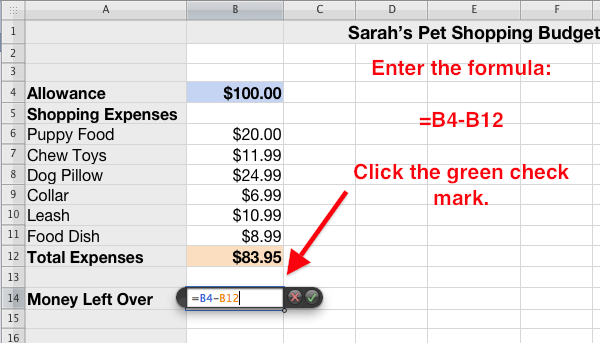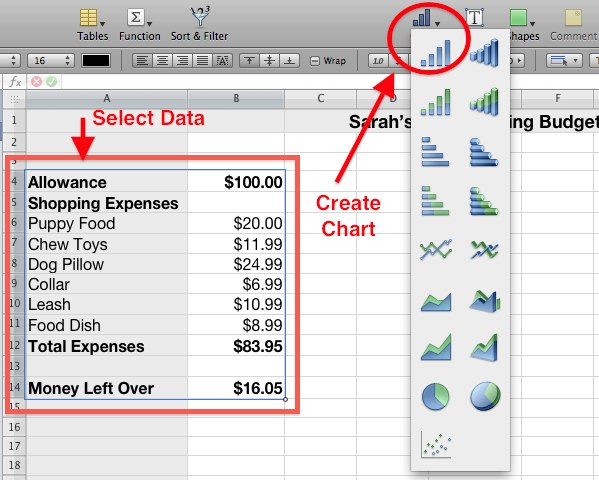# iWork Numbers - Shopping Budget with Chart

## Students Create a Shopping Budget that Calculates Total Expenses and Money Left Over

### Students utilize the following spreadsheet skills:

1) Format a chart title and merge cells.

2) Enter and format data and numbers.

3) Use formulas to sum total expenses and subtract total expenses from spending allowance.

4) Create and format a bar chart.

### Instructions:

Students begin with \$100.00 budget and enter 6 items to purchase for new pet. Students enter costs for each item, limiting their total to less than \$100.00.

Students use the Sum function to automatically calculate the total expenses. Students enter a formula to automatically subtract the \$100.00 spending allowance from the total expenses.

Students use the data to create a column chart. Students format the data with increased font sizes, bold text, dollar signs and chart colors.

## Finished iWork Numbers Shopping Budget Example:## Calculating the Total Expenses

To calculate the Total Expenses, click and drag to select all of the expenses. Next, click the function button and click Sum. This adds all of the expenses together and puts the total directly underneath.## Formula for Calculating Money Left Over

Click in the cell under the expenses and enter the equal sign. Enter the formula B4-B12. This subtracts the value in cell B4 from the value in B12. Click the green check mark to complete the calculation.## Creating the Shopping Budget Chart

Select the data as shown below. Click the Chart button and click the column chart selection.